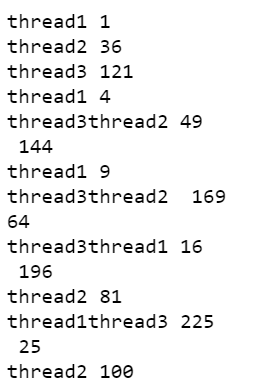Open in App
Not now

# How to run same function on multiple threads in Python?

• Difficulty Level : Medium
• Last Updated : 26 Mar, 2021

In a large real-world application, the modules and functions have to go through a lot of input and output-based tasks like reading or updating databases, communication with different micro-services, and request-response with clients or peers. These tasks may take a significant amount of time to complete.

The time taken in serving a request and responding to the client is called latency and programmers need to reduce latency as much as possible. This leads to the need for parallel processing where our application is able to execute some function or method with different parameters for different clients. We can achieve that using threading. A thread can execute a function in parallel with other threads. Each thread shares the same code, data, and files while they have their own stack and registers.

### Module Used:

In Python, we can create and run threads using the threading module. This module in python provides powerful and high-level support for threads.

### Step-by-step Approach:

• Import the libraries. We will use threading module to create and run thread. To observe the output, we will create some delay using time module.
```import threading
import time```
• Define a sample function that we will use to run on different threads. In this example lets make a function that prints the squares of numbers in the given list.
```# A sample function to print squares

for number in numbers:

# Produce some delay to see the output
# syntax: time.sleep(<time in seconds : float>)
time.sleep(1)```

```# Creating 3 threads that execute the same function with different parameters
target=print_squares, args=("thread1", [1, 2, 3, 4, 5]))

target=print_squares, args=("thread2", [6, 7, 8, 9, 10]))

target=print_squares, args=("thread3", [11, 12, 13, 14, 15]))```
• Now we need to start the execution. The Thread class has a start() method that transit the thread in running mode. The threads will run until they are not completed.
```# Start the threads
• We can block the program execution while all the threads are not completed using join() method of the Thread class.
```# Join the threads before moving further

Below is the full code:

## Python3

 `# Import module ` `import` `threading ` `import` `time ` ` `  ` `  `# A sample function to print squares ` `def` `print_squares(thread_name, numbers): ` `   `  `    ``for` `number ``in` `numbers: ` `        ``print``(thread_name, number``*``*``2``) ` `         `  `        ``# Produce some delay to see the output ` `        ``time.sleep(``1``) ` ` `  ` `  `# Creating 3 threads that execute the same  ` `# function with different parameters ` `thread1 ``=` `threading.Thread(target``=``print_squares,  ` `                           ``args``=``(``"thread1"``, [``1``, ``2``, ``3``, ``4``, ``5``])) ` ` `  `thread2 ``=` `threading.Thread(target``=``print_squares,  ` `                           ``args``=``(``"thread2"``, [``6``, ``7``, ``8``, ``9``, ``10``])) ` ` `  `thread3 ``=` `threading.Thread(target``=``print_squares,  ` `                           ``args``=``(``"thread3"``, [``11``, ``12``, ``13``, ``14``, ``15``])) ` ` `  `# Start the threads ` `thread1.start() ` `thread2.start() ` `thread3.start() ` ` `  `# Join the threads before  ` `# moving further ` `thread1.join() ` `thread2.join() ` `thread3.join() `

Output:My Personal Notes arrow_drop_up
Related Articles